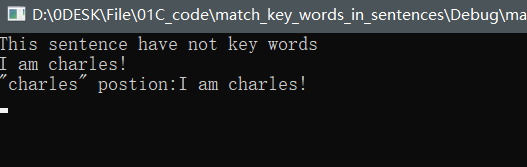﻿• 价格透明
• 信息保密
• 进度掌控
• 售后无忧# 【c语言笔记】字符串关键字匹配算法

Github代码下载地址：https://github.com/xiaocheng99/c_project

## 引言## 一、主函数

``````#include <stdio.h>
#define MAXLINE 1000

char pattern[] = "charles\0";
int main()
{
char charLine[MAXLINE];
int found = 0;
while (getLine(charLine, MAXLINE)>0)
{
//printf("keypress:%s", charLine);
if (strIndex(charLine,pattern)>=0)
{
printf("\"loud\" postion:%s", charLine);
found++;
}
}

return found;
}
``````

## 二、getLine函数

``````int getLine(char line[],int max)
{
int c, i;
i = 0;
while (--max > 0 && (c=getchar())!=EOF && c!= '\n')
{
line[i++] = c;
}
if (c=='\n')
{
line[i++] = c;
line[i] = '\0';
return i;

}
}
``````

## 三、strIndex函数

``````int strIndex(char source[],char serachfor[])
{
int i, j, k;
for (i = 0; source[i] != '\0'; i++)
{
for (j = i, k = 0; serachfor[k] != '\0'&&source[j] == serachfor[k];j++,k++);
if (k>0&&serachfor[k]=='\0')
{
return i;
}
}
return -1;
}
``````

Github代码下载地址：https://github.com/xiaocheng99/c_project### 低价透明### 金牌服务### 信息保密